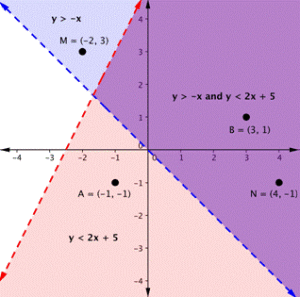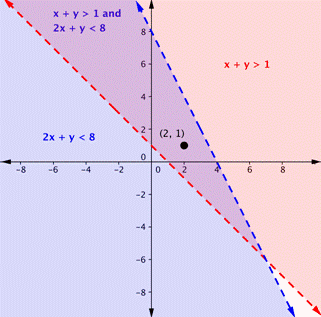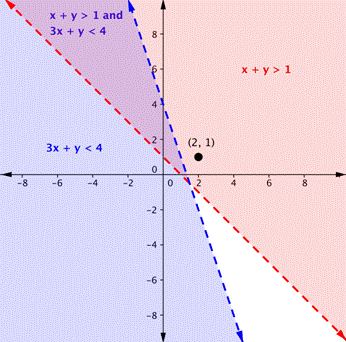## Defining Solutions to Systems of Linear Inequalities

### Learning Outcomes

• Identify solutions to systems of linear inequalities

Previously, we learned how we can plug an ordered pair into a system of equations to determine whether it is a solution to the system.  We can use this same method to determine whether a point is a solution to a system of linear inequalities.

## Determine whether an ordered pair is a solution to a system of linear inequalitiesOn the graph above, you can see that the points B and N are solutions for the system because their coordinates will make both inequalities true statements.

In contrast, points M and A both lie outside the solution region (purple). While point M is a solution for the inequality $y>−x$ and point A is a solution for the inequality $y<2x+5$, neither point is a solution for the system. The following example shows how to test a point to see whether it is a solution to a system of inequalities.

### Example

Is the point $(2, 1)$ a solution of the system $x+y>1$ and $2x+y<8$?

Here is a graph of the system in the example above. Notice that $(2, 1)$ lies in the purple area, which is the overlapping area for the two inequalities.### Example

Is the point $(2, 1)$ a solution of the system $x+y>1$ and $3x+y<4$?

Here is a graph of this system. Notice that $(2, 1)$ is not in the purple area, which is the overlapping area; it is a solution for one inequality (the red region), but it is not a solution for the second inequality (the blue region).You can watch the video below for another example of how to verify whether an ordered pair is a solution to a system of linear inequalities.

## Summary

• Solutions to systems of linear inequalities are entire regions of points.
• You can verify whether a point is a solution to a system of linear inequalities in the same way you verify whether a point is a solution to a system of equations.

## Contribute!

Did you have an idea for improving this content? We’d love your input.